﻿

### On derivable Baer-elation planes

#### Abstract

In , Jha and Johnson introduce Baer-elation planes. These are finite translation planes of order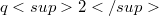$q2$,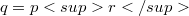$q=pr$ which admit both Baer p-collineation groups and elation groups which normalize each other.By a result of Foulser ,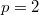$p=2$.Jha-Johnson consider, in particular, Baer-elation planes of order$q2$ with kernel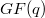$GF(q)$ of type (2,q) or type (q,2). That is, there is a Baer or elation group of order q.By the incompatibility results of Jha-Johnson , , the corresponding or Baer group has order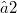$≤ 2$.

DOI Code: 10.1285/i15900932v7n1p19

Full Text: PDF